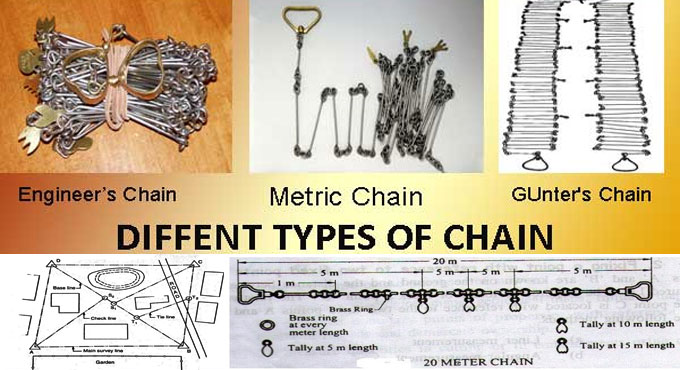# Tape Corrections in Chain SurveyingSurveying is often required in any field of engineering, civil is no different. Here, our main focus will be on chain surveying. This is a very old and simple method. This chain method is performed by a use of tape and it is expected to have errors due to incorrect tape measurements.

In any local chaining works, the errors are ignored. However, in highly sophisticated works accurate tape correction are provided. The correction is positive if the erroneous length is to be increased and the correction is negative if the erroneous length must be decreased.

There are seven common errors on which corrections are performed in linear measurement are: Correction for absolute length, Correction for pull or tension, Correction for temperature, Correction for Sag, Correction for Alignment, Correction for Slope, Reduction for the sea level.

Correction for Absolute Length:
Ca = L*c/l

Here, L = Measured length of the line; c = Correction per tape length; l = designated length of the tape or the nominal length.

Different cases are:

• Absolute length > Designated length means, measured distance is short, hence the correction is additive.
• Absolute length < Designated length means, measured distance is long, hence the correction is subtractive.

The sign of correction Ca is the same as that of ‘c’.

Correction for Pull or Tension:
The formula for the correction for pull or tension is given by:
Cp = {(P - Po) * L} / (A * E)

Where, P = Pull applied during the measurement; Po = Standard pull; Both P and Po are measured in Newton; L = measured length; A = area of cross-section in cm2; E = Young’s modulus in N/cm2.

1. The correction is additive when, pull applied during the measurement is greater than standard pull i.e.
P > Po
2. The correction is subtractive when, pull applied during measurement is less than standard pull.
P < Po
Correction for Temperature:
Correction for temperature Ct is given by:
Ct = α * (T m – T α) * L
Where, α = Coefficient of thermal expansion; Tm = mean temperature in the field during measurement; To = temperature during standardization; L = measured length.

Two possible cases:

• When the mean temperature in the field during temperature is greater than temperature during standardization i.e. Tm > To this results in an increase in the tape length, making the measured length shorter. Thus the result is an additive correction.
• When the mean temperature in the field is less than temperature during standardization i.e. Tm < To this results in an increase in the measured length than the original. Hence the correction is subtractive.

Correction for Sag: While measuring on unevenly sloping ground, tapes are suspended at shorter length and horizontal distances are measured. This technique eliminates errors due to measurement along slopes, but necessitates correction for sag. Hence, measured length is more than actual length. Thus the correction is negative. The correction, which is difference between the length of catenary and true length is given by:

Cs = (l * W2) / (24 * n2P2)
Where, Cs =tape correction per tape length; l = total length of the tape; W = total weight of the tape; n = number of equal spans; P = pull applied